# RD Sharma Solutions Class 9 Chapter 17 Construction

Read RD Sharma Solutions Class 9 Chapter 17 Construction below, students should study RD Sharma class 9 Mathematics available on Studiestoday.com with solved questions and answers. These chapter wise answers for class 9 Mathematics have been prepared by teacher of Grade 9. These RD Sharma class 9 Solutions have been designed as per the latest NCERT syllabus for class 9 and if practiced thoroughly can help you to score good marks in standard 9 Mathematics class tests and examinations

Exercise 17.1

Question 1: Draw a line segment of length 8.6 cm. Bisect it and measure the length of each part.
Solution: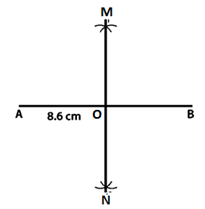(1) Draw a line segment with the length AB = 8.6 cm.
(2) Using A as a core, draw arcs on either side of AB at any radius greater than half of 8.6.
(3) Repeat Step 2 with B as the centre, making sure that these arcs intersect with the previous arcs.
(4) Connect the points M and N that cross AB at O.

Question 2: Draw a line segment AB of length 5.8cm. Draw the perpendicular bisector of this line segment.
Solution: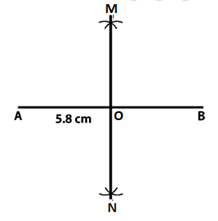(1) Draw a line segment with the length AB = 5.8 cm.
(2) Using A as a core, draw arcs on either side of AB at any radius greater than half of 5.8.
(3) Repeat Step 2 with B as the centre, making sure that these arcs intersect with the previous arcs.
(4) Connect the M and N points.

Question 3: Draw a circle with centre at point O and radius 5cm. Draw its chord AB, the perpendicular bisector of line segment AB. Does it pass through the centre of the circle?
Solution: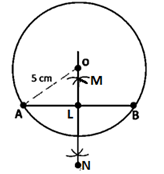(1) Draw a circle with a radius of 5 cm and a centre of point O.
(2) Using the scale, create an AB chord.
(3) Draw an arc on either side of the chord, with A as the centre and a radius of more than half of 5 cm.
(4) Using B as a core, repeat step 3 to make sure these arcs cut the previous arcs.
(5) Get M and N together.
Hence, MN is a perpendicular bisector of chord AB.

Question 4:  Draw a circle with centre at point O. Draw its two chords AB and CD such that AB is not parallel to CD. Draw the perpendicular bisectors of AB and CD. At what point do they intersect?
Solution: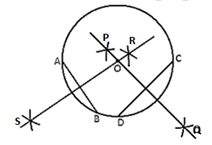(1) Draw a circle with O as the centre and some radius.
(2) Draw the AB and CD chords.
(3) Starting with A, draw arcs on either side of AB using more than half of AB.
(4) With B as the centre and the same radius, draw arcs that intersect the previous arcs at points R and S.
(5) Connect the R and S points.
(6) Draw arcs on either side of DC, with D as the centre and a radius greater than half of DC.
(7) With C as the centre and the same radius, draw arcs that intersect the previous arcs at points P and Q.
(8) Connect the P and Q points.

Question 5:  Draw a line segment of length 10 cm and bisect it. Further, bisect one of the equal parts and measure its length.
Solution:(1) Draw a 10-cm-lengthy line segment AB.
(2) Keeping A as the middle, draw arcs on either side of AB using a point that is more than half the length of AB.
(3) With B as the centre and the same radius, draw arcs that intersect the previous arc at points R and S.
(4) Join P and Q at C, where they intersect AB.
(5) Draw arcs on either side of AC with A as the middle and a radius greater than half of AC.
(6) With C as the centre and the same radius, draw arcs that intersect the previous arcs at points P and Q.
(7) Connect the points R and S, which cross AC at D.

Question 6:  Draw a line segment AB bisect it. Bisect one of the equal parts to obtain a line segment of length (1/4) (AB).
Solution: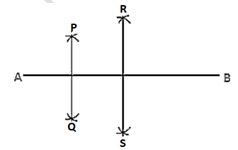(1) Begin by drawing a line segment AB.
(2) Draw arcs on either side of AB with A as the middle and a radius greater than half of AB.
(3) Using B as the centre and the same radius, draw arcs that intersect the previous arcs at points R and S.
(4) Join R and S at C, where they intersect AB.
(5) Draw two circles, one on either side of AC, with A as the centre and radius.
(6) Draw arcs at P and Q, with C as the middle and the same radius as the previous arcs.
(7) Connect the points R and S, which cross AC at D.
As a result, the line AD is one-fourth of the line AB.

Question 7:  Draw a line segment AB and by ruler and compasses, obtain a line segment of length (3/4)(AB).
Solution: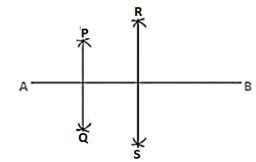1. Begin by drawing a line segment AB.
2. Draw arcs on either side of AB with A as the middle and a radius greater than half of AB.
3. Using B as the centre and the same radius, draw arcs that intersect the previous arcs at points R and S.
4. Join P and Q at C, where they intersect AB.
5. Draw two circles, one on either side of AC, with A as the centre and radius.
6. Draw arcs at P and Q, with C as the middle and the same radius as the previous arcs.
7. Connect the points R and S, which cross AC at D.
As a result, the line AD is three-quarters of the line AB.

Exercise 17.2

Question 1: Draw an angle and label it as ∠BAC. Construct another angle, equal to ∠BAC.Solution:
(1) Draw a line section QR and an angle ABC.
(2) Draw an arc with centre A and any radius that intersects BAC at M and N.
(3) Draw an arc of the same radius as Q that intersects QR at S.
(4) Draw an arc with S as the centre and DE as the radius that intersects the previous arc at T.
(5) Connect Q and T with a line section.
As a result, ∠PQR = ∠BAC.

Question 2: Draw an obtuse angle. Bisect it. Measure each of the angles so formed.
Solution: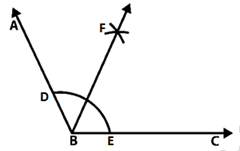(1) Draw a 120° angle ABC.
(2) Draw an arc with B as the centre and any radius that intersects AB at P and BC at Q.
(3) Draw an arc with D as the origin and a radius greater than half of DE.
(4) Draw an arc with E as the middle and the same radius as the previous arc, cutting it at R.
(5) Connect B to R.
As a result, ∠ABR = ∠RBC = 60°.

Question 3: Using your protractor, draw an angle of 108°. With this given angle as given, draw an angle of 54°.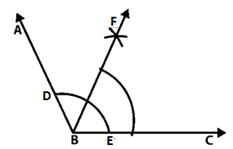Solution:
(1) Draw ∠ABC = 108° on a piece of paper.
(2) From point B, draw an arc that intersects AB at D and BC at E. (Select any radius)
(3) From point D, draw an arc with a radius greater than half of DE.
(4) Repeat Step 3 with E as the centre and the previous arc intersected at F.
(5) Connect B to F.
As a result, ∠FBC = 54°.

Question 4: Using the protractor, draw a right angle. Bisect it to get an angle of measure 45°.
Solution:(1) Construct ∠ABC at a 90°
(2) From point B, draw an arc that intersects AB at D and BC at E. (Select any radius)
(3) From point D, draw an arc with a radius greater than half of DE.
(4) Repeat step 3 with E as the centre and the previous arc intersected at F.
(5) Join F to B.
As a result, ∠RBC=45°.

Question 5:  Draw a linear pair of angles. Bisect each of the two angles. Verify that the two bisecting rays are perpendicular to each other.
Solution:(1) Create a linear pair by drawing two angles DCA and DCB.
(2) Using any radius and centre C, draw an arc that intersects AC at P, CD at Q, and CB at R.
(3) Using any radius and centre P and Q, draw two arcs that intersect at S.
(4) Join S to C.
(5) Draw two arcs that converge at T, with Q and R as the centres and some radius.
(6) Join T to C.
As a result, SCT = 90°.

Question 6:  Draw a pair of vertically opposite angles. Bisect each of the two angles. Verify that the bisecting rays are in the same line.
Solution:(1) Construct ∠AOC and ∠DOB, two vertically opposite angles.
(2) Draw two arcs with O as the middle and some radius, intersecting OA at P, OC at Q, OB at S, and OD at R.
(3) Draw two arcs that converge at T, with P and Q as the centres and a radius greater than half of PQ.
(4) Join T to O.
(5) Draw two arcs that converge at U, keeping R and S as centres and a radius greater than half of RS.
(6) Join O to U.
As a result, ∠TOU is a straight line.

Question 7:  Using rulers and compasses only, draw a right angle.
Solution:(1) Begin by drawing a line segment AB.
(2) Using any radius and keeping A as the base, draw an arc that intersects AB at C.
(3) Draw an arc that intersects the previous arc at D, keeping C as the middle and the same radius.
(4) Draw an arc that intersects the arc in (2) at E, keeping D as the middle and the same radius.
(5) Draw arcs that converge at F, keeping E and D as the centre and a radius greater than half of ED.
(6) Join F to A.
As a result, FAB = 90°.

Question 8:  Using rulers and compasses only, draw an angle of measure 135°.
Solution:(1) Draw a line segment AB and generate BA to C.
(2) Keeping A as the centre and any radius draw an arc which intersects AC at D and AB at E.
(3) Draw arcs that converge at F, keeping D and E as centres and a radius greater than half of DE.
(4) Join FA at G, where it intersects the arc in (2).
(5) Draw arcs that converge at H, keeping G and D as the centre and a radius greater than half of GD.
(6) Becoming a member of HA.
As a result, ∠HAB = 135°.

Question 9:  Using a protractor, draw an angle of measure 72°. With this angle as given draw angles of measure 36° and 54°.
Solution: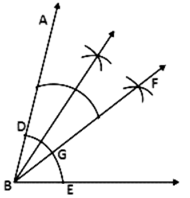(1) Using a protractor, draw an ∠ABC of 72 degrees.
(2) Using any radius and B as the base, draw an arc that intersects AB at D and BC at E.
(3) Draw two arcs that converge at F, with D and E as the centres and a radius greater than half of DE.
(4) Join FB at G, where it intersects the arc in (2).
(5) Draw two arcs that converge at H, with D and G as the centres and a radius greater than half of DG.
(6) Join H to B.
As a result, ∠HBC = 54° and ∠FBC = 36°.

Question 10:  Construct the following angles at the initial point of a given ray and justify the construction:
(i) 45°
(ii) 90°
Solution: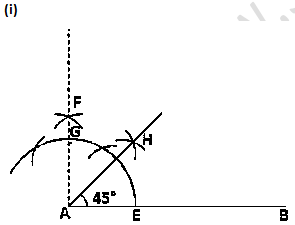(1) Generate BA to C by drawing a line section AB.
(2) Using any radius and keeping A as the base, draw an arc that intersects AC at D and AB at E.
(3) Draw arcs that converge at F, keeping D and E as the centre and a radius greater than half of DE.
(4) Join FA at G, where it intersects the arc in (2).
(5) Draw arcs that intersect at H, keeping G and E as the centre and radius more than half of GE.
(6) Join H to A.
As a result, ∠HAB = 45°.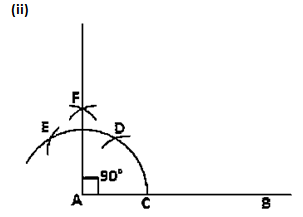(1) Begin by drawing a line segment AB.
(2) Using any radius and keeping A as the base, draw an arc that intersects AB at C.
(3) Draw an arc that intersects the previous arc at D, keeping C as the middle and the same radius.
(4) Draw an arc that intersects the arc in (2) at E, keeping D as the middle and the same radius.
(5) Draw arcs that converge at F, keeping E and D as the centre and a radius greater than half of ED.
(6) Join F to A.
As a result, FAB = 90°.

Question 11:  Construct the angles of the following measurements:
(i) 30°
(ii) 75°
(iii) 105°
(iv) 135°
(v) 15°
(vi) 22 1/2°
Solution: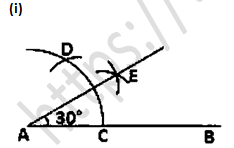(1) Begin by drawing a line segment AB.
(2) Using any radius and keeping A as the base, draw an arc that intersects AB at C.
(3) Draw an arc that intersects the previous arc at D, keeping C as the middle and the same radius.
(4) Draw arcs that converge at E using D and C as centres and a radius greater than half of DC.
(5) Join point E to point A.
As a result, EAB = 30°.(1) Begin by drawing a line segment AB.
(2) Using any radius and keeping A as the base, draw an arc that intersects AB at C.
(3) Draw an arc that intersects the previous arc at D, keeping C as the middle and the same radius.
(4) Draw an arc that intersects the arc in (2) at E, keeping D as the middle and the same radius.
(5) Draw arcs intersecting at F, keeping E and D as the centre and the radius more than half of ED.
(6) At G, join FA, which intersects the arc in (2).
(7) Draw arcs intersecting at H with G and D as the base and a radius greater than half of GD.
(8) Join point H to A.
As a result, HAB = 75°.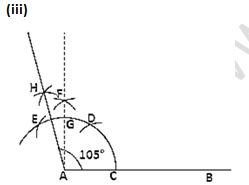(1) Begin by drawing a line segment AB.
(2) Using any radius and keeping A as the base, draw an arc that intersects AB at C.
(3) Draw an arc that intersects the previous arc at D, keeping C as the middle and the same radius.
(4) Draw an arc that intersects the arc in (2) at E, keeping D as the centre and the same radius.
(5) Draw arcs that converge at F, keeping E and D as the centre and a radius greater than half of ED.
(6) Join FA at G, where it intersects the arc in (2).
(7) Draw arcs that converge at H, with E and G as the centres and a radius of more than half of EG.
(8) Join point H to A.
As a result, ∠HAB = 105°.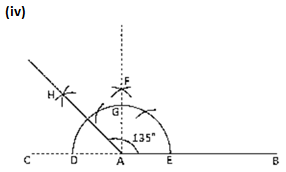(1) Generate BA to C by drawing a line segment AB.
(2) Using any radius and keeping A as the base, draw an arc that intersects AC at D and AB at E.
(3) Draw arcs that converge at F, keeping D and E as centres and a radius greater than half of DE.
(4) Join FA at G, where it intersects the arc in (2).
(5) Draw arcs that converge at H, keeping G and D as centres and a radius greater than half of GD.
(6) Join point H to A.
As a result, ∠HAB = 135°.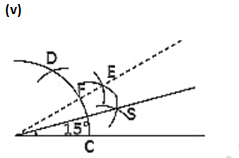(1) Begin by drawing a line segment AB.
(2) Using any radius and keeping A as the base, draw an arc that intersects AB at C.
(3) Draw an arc that intersects the previous arc at D, keeping C as the middle and the same radius.
(4) Draw arcs that converge at E, using D and C as centres and a radius of more than half of DC.
(5) Join EA at F, where it intersects the arc in (2).
(6) With F and C as the centres and a radius of more than half of FC, draw arcs that converge at G.
(7) Join point G to A.
As a result, ∠GAB = 15°.(1) Draw the AB line section.
(2) Draw an arc that intersects AB at C with A as the middle and some radius.
(3) Draw an arc that intersects the previous arc at D, keeping C as the middle and the same radius.
(4) Draw an arc that intersects the arc in (2) at E, keeping D as the centre and the same radius.
(5) Draw arcs that converge at F, keeping E and D as the centre and a radius of more than half of ED.
(6) At G, join FA, which intersects the arc in (2).
(7) Draw arcs intersecting each other at point H, keeping G and C as the centre and radius more than half of GC.
(8) Join HA at point I, which intersects the arc in (2).
(9) Draw arcs intersecting at point J, keeping I and C as centres and a radius greater than half of IC.
(10) Join point J to A.
As a result, ∠JAB = 22 1/2° .

Exercise 17.3

Question 1: Construct a △ABC in which BC = 3.6 cm, AB + AC = 4.8 cm and ∠B = 60°.
Solution:(1) Draw a BC = 3.6 cm line segment.
(2) Draw ∠XBC = 60° at the stage B.
(3) Draw an arc with a radius of 4.8 cm that intersects XB at point D from point B.
(4) Join D to C.
(5) Draw a perpendicular bisector of DC that passes through A and intersects DB.
As a result, the requisite triangle is ∆ABC.

Question 2: Construct a △ABC in which AB + AC = 5.6 cm, BC = 4.5 cm and ∠B=45°.
Solution: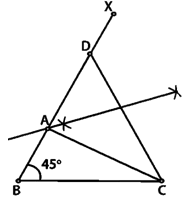(1) Draw a BC = 4.5 cm line segment.
(2) Draw XBC = 45° at the stage B.
(3) Draw an arc with a radius of 5.6 cm that intersects XB at point D from point B.
(4) Join D to C.
(5) Draw a perpendicular bisector of DC that passes through A and intersects DB.
(6) Join A to C.
As a result, the requisite triangle is ∆ABC.

Question 3: Construct a △ABC in which BC = 3.4 cm, AB – AC = 1.5 cm and ∠B = 45°.
Solution: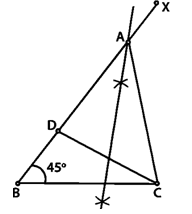(1) Draw a BC = 3.4 cm line segment.

(2) Draw ∠XBC = 45°.

(3) Draw an arc with a radius of 1.5 cm that intersects XB at point D from point B. As a result, BD = 1.5 cm.

(4) Enter the DC line section.

(5) Draw a perpendicular bisector of DC that passes through A and intersects BX.

(6) Enter the AC line section.

As a result, the requisite triangle is ∆ABC.

Question 4: Using rulers and compasses only, construct a △ABC, given base BC = 7 cm, ∠ABC = 60° and AB + AC = 12 cm.

Solution:(1) Draw a BC = 7 cm line segment.

(2) Draw an arc from point B to point N, cutting BC. (You can choose any radius.)

(3) With the same radius as in step 2, keep the compass at point N and sever the previous arc at M.

(4) Join BM line section.

(5) Make BM at some stage P.

(6) From BP, cut BR = 12 cm.

(7) Becoming a member of CR.

(8) Draw a perpendicular bisector of RC that passes through A and intersects BR.

(9) Join line section AC together.

As a result, the requisite triangle is ABC.

Question 5:  Construct a triangle whose perimeter is 6.4 cm, and angles at the base are 60° & 45°.

Solution: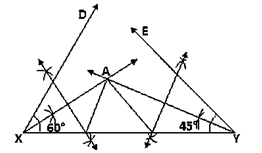(1) Construct a line segment XY of 6.4 cm.
(2) Construct ∠DXY = 60° and ∠EYX = 45°.
(3) Construct the angle bisectors of DXY and EYX where they converge at A.
(4) Construct the AX and AY perpendicular bisectors that intersect XY at B and C, respectively.
(5) Assemble AB and AC.
As a result, the requisite triangle is ABC.

Question 6:  Using rulers and compasses only, construct a △ABC from the following data: AB + BC + CA = 12 cm, ∠B = 45° and ∠C = 60°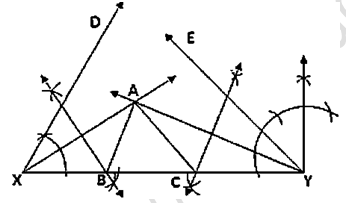Solution:
(1) Construct a line segment XY of 12 cm.
(2) Construct ∠DXY = 45° and ∠EYX = 60°.
(3) Construct the angle bisectors of DXY and EYX where they converge at A.
(4) Construct the AX and AY perpendicular bisectors that intersect XY at B and C, respectively.
(5) Join A to B and A to C.
As a result, the requisite triangle is ABC.

Question 7:  Construct a right-angled triangle whose perimeter is equal to 10 cm and one acute angle equal to 60°.
Solution: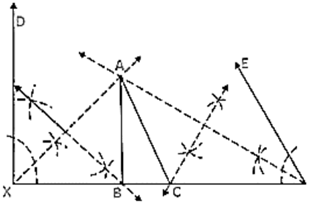(1) Draw a 10 cm XY line segment.
(2) Draw DXY = 90 degrees and EYX = 60 degrees.
(3) Draw the angle bisectors of DXY and EYX where they converge at A.
(4) Draw the AX and AY perpendicular bisectors that intersect XY at B and C, respectively.
(5) Assemble AB and AC.
As a result, the requisite triangle is ABC.

Question 8:  Construct a triangle ABC such that BC = 6 cm, AB = 6 cm and median AD = 4 cm.
Solution:1. Draw a 6 cm BC line segment.
2. Take the mid-point O of the BC line.
3. Draw two arcs that converge at A, with centres B and D and radii of 6cm and 4cm.
4. Assemble AB, AD, and AC.
As a result, the requisite triangle is ABC.

Question 9:  Construct a right-angled triangle ABC whose base BC is 6 cm and the sum of the hypotenuse AC and other side AB is 10 cm.
Solution: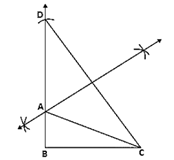(1) Create a 6cm-long BC line section.
(2) Draw XBC = 90° at the stage B.
(3) Draw an arc that intersects XB at D, with B as the middle and a radius of 10cm.
(4) Becoming a DC member.
(5) Draw the perpendicular bisector of DC that passes through A and intersects DB.
(6) Join A to C.
As a result, the requisite triangle is ABC.

Question: 10 Construct a triangle XYZ in which ∠Y = 30°, ∠Z = 90° and XY + YZ + ZX = 11 cm.
Solution: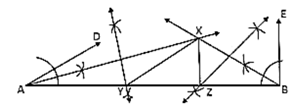(1) Draw an 11cm line section AB.
(2) Draw DAB at 30 degrees and FBA at 90 degrees.
(3) Draw the angle bisectors of DAB and EBA at X, where they converge.
(4) Draw the perpendicular bisector of XA and XB that intersects AB at Y and Z.
(5) Team up with XY and XZ.
As a result, the necessary triangle is XYZ.